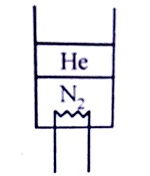$∆H$ for the reaction 2C(s) + 3H2(g)$\to$C2H6(g) is -20.24 kcal/mol. To what value of the enthalpy of sublimation of C(s) does this point given that the bond energies of C-C, C-H and H-H are 63 kcal/mol, 85.6 kcal/mol and 102.6 kcal/mol.

1. 124.3 kcal/mol

2. 185.3 kcal/mol

3. 158.3 kcal/mol

4. 211.5 kcal/mol

High Yielding Test Series + Question Bank - NEET 2020

Difficulty Level:

The gas absorbs 100 J heat and is simultaneously compressed by a constant external pressure of 1.50 atm from 8 lit. to 2 lit. in volume. Hence $∆E$ will be-

1. -812 J

2. 812 J

3. 1011 J

4. 911 J

High Yielding Test Series + Question Bank - NEET 2020

Difficulty Level:

If $∆G=∆H-T∆S$ and $∆G=∆H+T{\left(\frac{d\left(∆G\right)}{dT}\right)}_{P}$ then variation of EMF of a cell E, with temperature T, is given by

1. $\frac{∆H}{nF}$

2. $\frac{∆G}{nF}$

3. $\frac{∆S}{nF}$

4. $-\frac{∆S}{nF}$

High Yielding Test Series + Question Bank - NEET 2020

Difficulty Level:

The standard heat of combustion of Al is -837.8 kJ mol-1 at ${25}^{\circ }C$ which of the following releases 250 kcal of heat ?

1. The reaction of 0.624 mol of Al

2. The formation of 0.624 mol of Al2O3

3. The reaction of 0.312 mol of Al

4. The formation of 0.150 mol of Al2O3

High Yielding Test Series + Question Bank - NEET 2020

Difficulty Level:

${C}_{P}-{C}_{V}=R$. This R is :

1. Change in K.E.

2. Change in rotational energy

3. work done which system can do on expanding the gas per mol per degree increase in temperature

4. All correct

High Yielding Test Series + Question Bank - NEET 2020

Difficulty Level:

Heat of neutralisation of oxalic acid is -25.4 K cal mol-1 using strong base, NaOH. Hence enthalpy change of the process is is-

1. 2.0 kcal

2. -11.8 kcal

3. 1.0 kcal

4. -1.0 kcal

High Yielding Test Series + Question Bank - NEET 2020

Difficulty Level:

5 moles of nitrogen gas are enclosed in an adiabatic cylindrical vessel. The piston itself is a rigid light cylindrical container containing 3 moles of Helium gas. There is a heater which gives out a power 100 cal to the nitrogen gas. A power of 30 cal is transferred to Helium through the bottom surface of the piston.

The rate of increment of temperature of the nitrogen gas assuming that the piston moves slowly :1. 2K/sec

2. 4K/sec

3. 6K/sec

4. 8K/sec

Concept Questions :-

CP and CV and Relation for Real Gas
High Yielding Test Series + Question Bank - NEET 2020

Difficulty Level:

During the isothermal mixing of ideal gases at pressure, p, the entropy change per mole for the mixing process is-R  where x1, x2,....,xi are the mole fractions of the components, 1, 2,....,i of the mixture. Assuming ideal gas behavior, calculate $∆S$ for the mixing of 0.8 mole of N2 and 0.2 mole of O2.(at ${25}^{\circ }C$ and 0.9 atm) [1 eu = cal/deg]

1. 0.9943 eu

2. 0.7533 eu

3. 0.6798 eu

4. 0.7112 eu

Concept Questions :-

Spontaneity and Entropy
High Yielding Test Series + Question Bank - NEET 2020

Difficulty Level:

An ideal gas can be expanded from an initial state to a certain volume through two different processes, (A) $P{V}^{2}=K$ (B) $P=K{V}^{2}$, where K is a positive constant. Then, choose the correct option from the following.

1. Final temperature in (A) will be greater than in (B)

2. Final temperature in (B) will be greater than in (A)

3. Work done by the gas in both the processes would be equal

4. Total heat given to the gas in (A) is greater than in (B)

Concept Questions :-

Extensive and Intensive Properties
High Yielding Test Series + Question Bank - NEET 2020

Difficulty Level:

For the reaction :

1. 2.7 kcal

2. -2.7 kcal

3. 9.3 kcal

4. -9.3 kcal

Concept Questions :-

Gibbs Energy Change